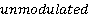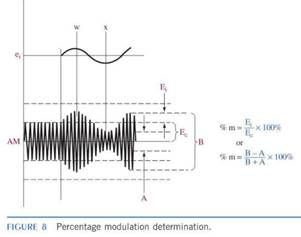Create an Account

Home / Questions / 1 Draw a diagram of a carrier wave envelope when modulated 50 percent by a sinusoidal wave...

1 Draw a diagram of a carrier wave envelope when modulated 50 percent by a sinusoidal wave Indicate on the diagram the dimensions from which the percentage of modulation is determined 2 What are

1. Draw a diagram of a carrier wave envelope when modulated 50 percent by a sinusoidal wave. Indicate on the diagram the dimensions from which the percentage of modulation is determined.

2. What are some of the possible results of over modulation?

3. Ancarrier is 300 V p-p. Calculate %m when its maximum p-p value reaches 400, 500, and 600 V. (33.3%, 66.7%, 100%)

4. If A = 60 V and B = 200 Vas shown in Figure 8, determine %m. (53.85%)Jul 29 2020 View more View LessSubscribe To Get Solution